Courses

Test: Butterworth Filters Design - 1

10 Questions MCQ Test Digital Signal Processing | Test: Butterworth Filters Design - 1

Description
Attempt Test: Butterworth Filters Design - 1 | 10 questions in 10 minutes | Mock test for Electrical Engineering (EE) preparation | Free important questions MCQ to study Digital Signal Processing for Electrical Engineering (EE) Exam | Download free PDF with solutions
QUESTION: 1

The cutoff frequency of the low pass Butterworth filter is the arithmetic mean of the two cutoff frequencies as found above.

Solution:

The arithmetic mean of the two cutoff frequencies as found above is the final cutoff frequency of the low pass Butterworth filter.

QUESTION: 2

Which of the following is a frequency domain specification?

Solution:
• We are required to design a low pass Butterworth filter to meet the following frequency domain specifications.
• KP ≤ 20 log|H(jΩ)| ≤ 0 and 20 log|H(jΩ)| ≤ KS.
QUESTION: 3

What is the value of gain at the pass band frequency, i.e., what is the value of KP?

Solution:

We know that the formula for gain is: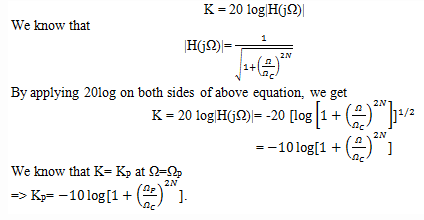QUESTION: 4

What is the value of gain at the stop band frequency, i.e., what is the value of KS?

Solution:

We know that the formula for gain is: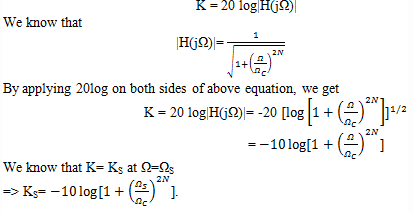QUESTION: 5

Which of the following equation is true?

Solution:

We know that,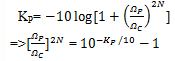QUESTION: 6

Which of the following equation is true?

Solution:

We know that: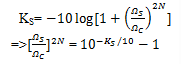QUESTION: 7

What is the order N of the low pass Butterworth filter in terms of KP and KS?

Solution:

Explanation: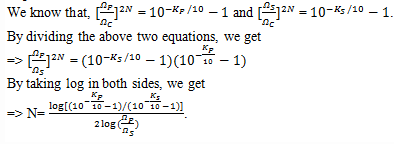QUESTION: 8

What is the expression for cutoff frequency in terms of pass band gain?

Solution:

We know that: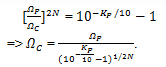QUESTION: 9

What is the expression for cutoff frequency in terms of stop band gain?

Solution:

We know that: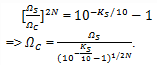QUESTION: 10

What is the lowest order of the Butterworth filter with a pass band gain KP= -1 dB at ΩP= 4 rad/sec and stop band attenuation greater than or equal to 20dB at ΩS= 8 rad/sec?

Solution:

We know that the equation for the order of the Butterworth filter is given as: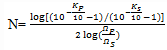From the given question:
KP= -1 dB, ΩP= 4 rad/sec, KS= -20 dB and ΩS= 8 rad/sec
Upon substituting the values in the above equation, we get N = 4.289
Rounding off to the next largest integer, we get N=5.Use Code STAYHOME200 and get INR 200 additional OFF Use Coupon Code

Track your progress, build streaks, highlight & save important lessons and more!

Similar ContentRelated tests